## Audio

[Audio][slideshow]

For the conversion of one flip flop to another, a combinational circuit has to be designed first. If a JK Flip Flop is required, the inputs are given to the combinational circuit and the output of the combinational circuit is connected to the inputs of the actual flip flop. Thus, the output of the actual flip flop is the output of the required flip flop. In this post, the following flip flop conversions will be explained.

• SR Flip Flop to JK Flip Flop
• JK Flip Flop to SR Flip Flop
• SR Flip Flop to D Flip Flop
• D Flip Flop to SR Flip Flop
• JK Flip Flop to T Flip Flop
• JK Flip Flop to D Flip Flop
• D Flip Flop to JK Flip Flop
• SR Flip Flop to JK Flip Flop

As told earlier, J and K will be given as external inputs to S and R. As shown in the logic diagram below, S and R will be the outputs of the combinational circuit.

The truth tables for the flip flop conversion are given below. The present state is represented by Qp and Qp+1 is the next state to be obtained when the J and K inputs are applied.

For two inputs J and K, there will be eight possible combinations. For each combination of J, K and Qp, the corresponding Qp+1 states are found. Qp+1 simply suggests the future values to be obtained by the JK flip flop after the value of Qp. The table is then completed by writing the values of S and R required to get each Qp+1 from the corresponding Qp. That is, the values of S and R that are required to change the state of the flip flop from Qp to Qp+1 are written.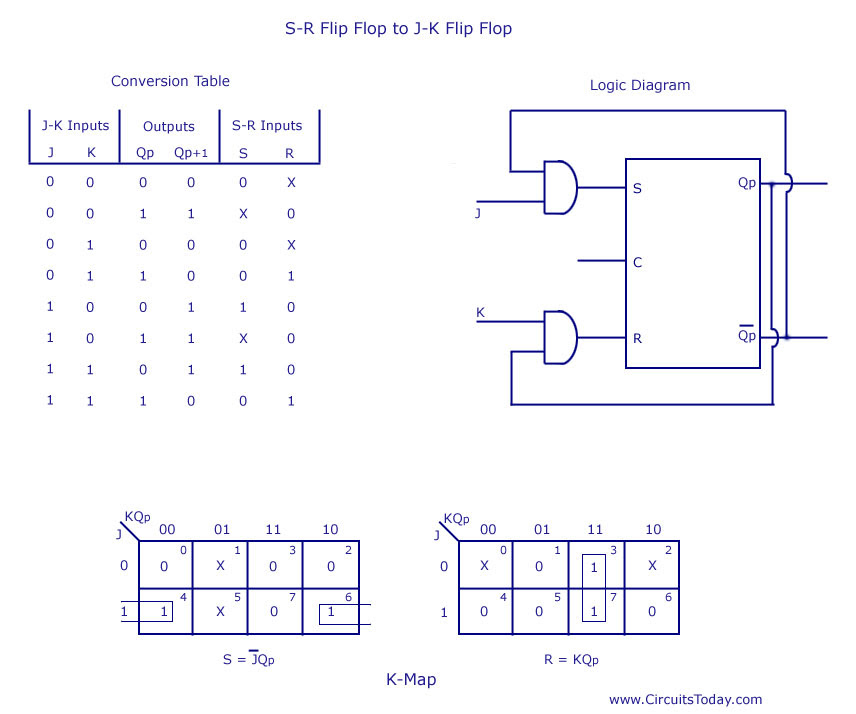SR Flip Flop to JK Flip Flop
• JK Flip Flop to SR Flip Flop

This will be the reverse process of the above explained conversion. S and R will be the external inputs to J and K. As shown in the logic diagram below, J and K will be the outputs of the combinational circuit. Thus, the values of J and K have to be obtained in terms of S, R and Qp. The logic diagram is shown below.

A conversion table is to be written using S, R, Qp, Qp+1, J and K. For two inputs, S and R, eight combinations are made. For each combination, the corresponding Qp+1 outputs are found ut. The outputs for the combinations of S=1 and R=1 are not permitted for an SR flip flop. Thus the outputs are considered invalid and the J and K values are taken as “don’t cares”.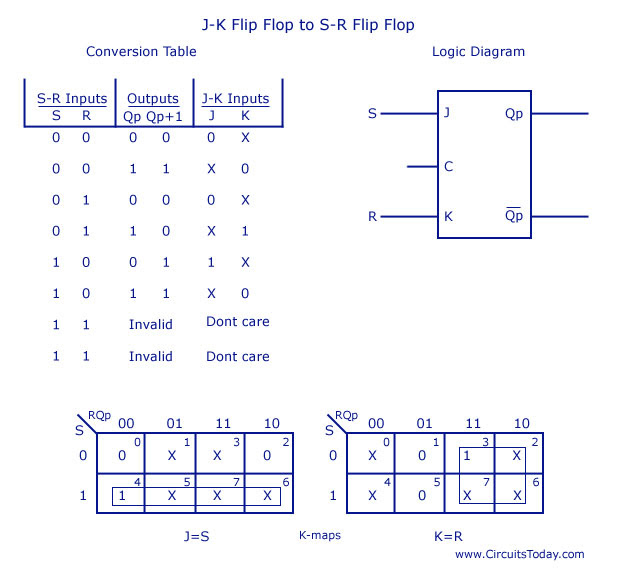JK Flip Flop to SR Flip Flop
• SR Flip Flop to D Flip Flop

As shown in the figure, S and R are the actual inputs of the flip flop and D is the external input of the flip flop. The four combinations, the logic diagram, conversion table, and the K-map for S and R in terms of D and Qp are shown below.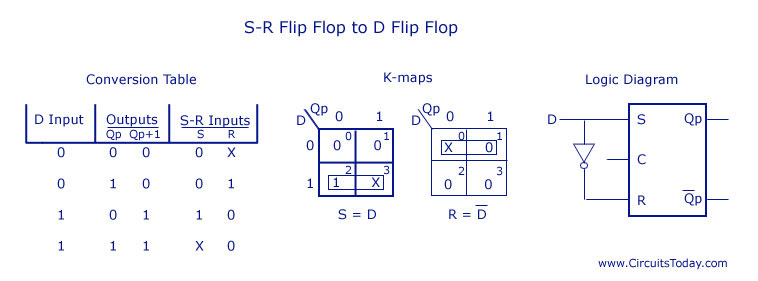SR Flip Flop to D Flip Flop
• D Flip Flop to SR Flip Flop

D is the actual input of the flip flop and S and R are the external inputs. Eight possible combinations are achieved from the external inputs S, R and Qp. But, since the combination of S=1 and R=1 are invalid, the values of Qp+1 and D are considered as “don’t cares”. The logic diagram showing the conversion from D to SR, and the K-map for D in terms of S, R and Qp are shown below.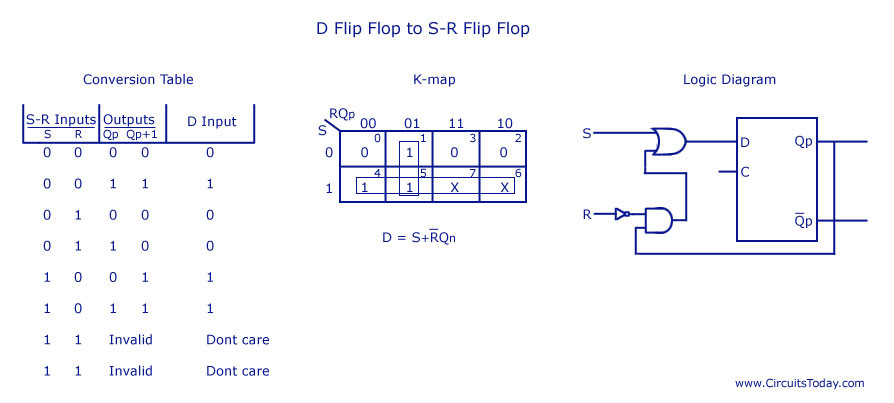D Flip Flop to SR Flip Flop
• JK Flip Flop to T Flip Flop

J and K are the actual inputs of the flip flop and T is taken as the external input for conversion. Four combinations are produced with T and Qp. J and K are expressed in terms of T and Qp. The conversion table, K-maps, and the logic diagram are given below.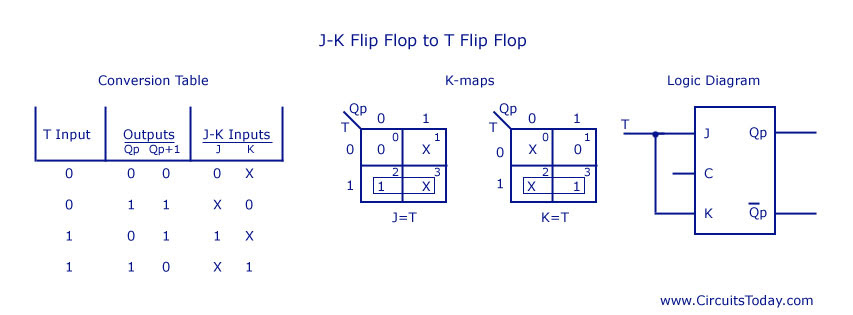JK Flip Flop to T Flip Flop
• JK Flip Flop to D Flip Flop

D is the external input and J and K are the actual inputs of the flip flop. D and Qp make four combinations. J and K are expressed in terms of D and Qp. The four combination conversion table, the K-maps for J and K in terms of D and Qp, and the logic diagram showing the conversion from JK to D are given below.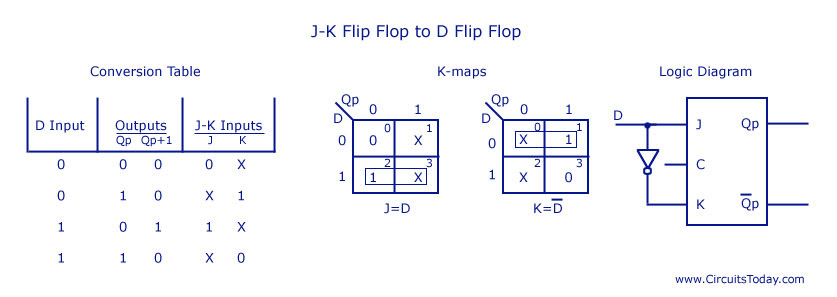JK Flip Flop to D Flip Flop
• D Flip Flop to JK Flip Flop

In this conversion, D is the actual input to the flip flop and J and K are the external inputs. J, K and Qp make eight possible combinations, as shown in the conversion table below. D is expressed in terms of J, K and Qp.

The conversion table, the K-map for D in terms of J, K and Qp and the logic diagram showing the conversion from D to JK are given in the figure below.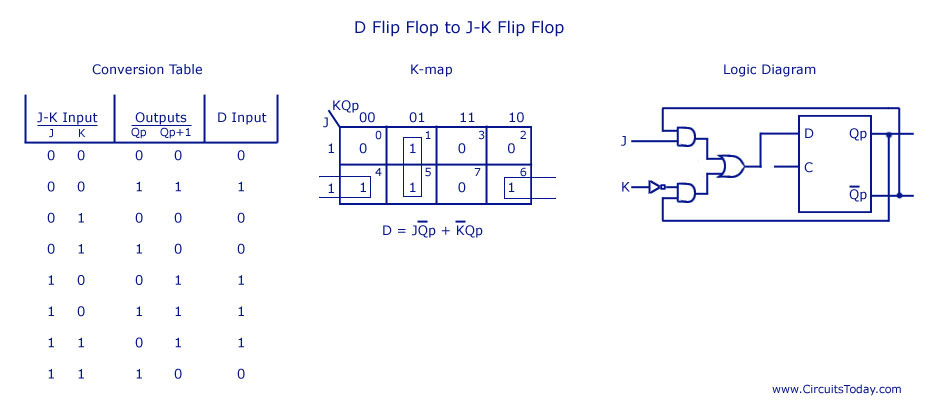D Flip Flop to JK Flip Flop

[Lights][grids]